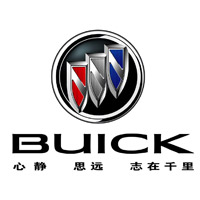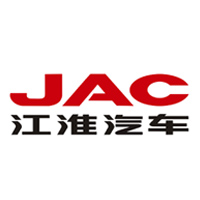• 选择品牌
选择品牌

• 选择车型

4.18将上市一款不可错过的新能源车东风悦达起亚河南普泽6S旗舰店

0371-86521188河南宇之林汽车销售服务有限公司

0371-55634444郑州市金裕通汽车销售服务有限公司

0371—63832666河南郑东天道长安福特4S旗舰店

0371-85333002郑州郑德宝汽车销售服务有限公司

4000663368-6268东风风行菱智 2015款 V3 1.5L 5座特供型 4.99万 4.99万 河南伟业东风风行4S店 询底价 比车价北汽绅宝绅宝D20 2015款 两厢 1.3L 手动乐活版 4.88万 4.88万 河南大广 询底价 比车价奔驰(进口)奔驰SLK级 2015款 SLK 200 碳纤维限量版 1.80万 1.80万 郑州之星 询底价 比车价江淮悦悦 2013款 国际版 1.0L 导航型 4.48万 4.48万 世纪阳光江淮 询底价 比车价海马汽车丘比特 2015款 1.3L 手动青葱版 4.99万 4.99万 http://zzglx.zhongbuauto.com/ 询底价 比车价东南V3菱悦 2014款 1.5L 手动精明版 4.98万 4.98万 郑州天翼汽车服务有限公司 询底价 比车价野马汽车野马F12 2014款 1.5L 手动 4.98万 4.98万 野马汽车河南精功 询底价 比车价

﻿
• 快速找车
• 选择品牌
• 选择品牌
• A  奥迪
• A  阿斯顿·马丁
• A  阿尔法·罗密欧
• B  宝沃
• B  布加迪
• B  巴博斯
• B  保时捷
• B  宾利
• B  奔驰
• B  宝马
• B  本田
• B  别克
• B  标致
• B  比亚迪
• B  宝骏
• B  北汽制造
• B  北汽新能源
• B  北汽幻速
• B  北汽威旺
• B  北京汽车
• B  奔腾
• B  北汽绅宝
• C  长安
• C  长安商用
• C  长城
• C  昌河
• D  大众
• D  道奇
• D  DS
• D  东南
• D  东风风神
• D  东风风行
• D  东风小康
• D  东风风度
• D  东风
• F  福特
• F  丰田
• F  菲亚特
• F  法拉利
• F  福田
• F  福迪
• F  福汽启腾
• G  观致
• G  广汽传祺
• G  广汽吉奥
• G  GMC
• H  红旗
• H  汉腾汽车
• H  哈弗
• H  哈飞
• H  海格
• H  海马
• H  华颂
• H  黄海
• H  华泰
• H  恒天
• J  吉利汽车
• J  捷豹
• J  Jeep
• J  江淮
• J  江铃
• J  金杯
• J  九龙
• J  金旅
• K  凯翼
• K  凯迪拉克
• K  克莱斯勒
• K  科尼塞克
• K  卡威
• K  开瑞
• L  路虎
• L  林肯
• L  劳斯莱斯
• L  兰博基尼
• L  雷克萨斯
• L  铃木
• L  雷诺
• L  理念
• L  力帆
• L  莲花汽车
• L  猎豹
• L  路特斯
• L  陆风
• M  马自达
• M  MG
• M  MINI
• M  玛莎拉蒂
• M  摩根
• M  迈凯轮
• N  纳智捷
• O  欧宝
• O  讴歌
• O  欧朗
• Q  奇瑞
• Q  起亚
• Q  启辰
• R  日产
• R  荣威
• R  瑞麒
• S  三菱
• S  斯威汽车
• S  萨博
• S  smart
• S  斯柯达
• S  斯巴鲁
• S  思铭
• S  双龙
• S  上汽大通
• S  双环
• T  特斯拉
• T  腾势
• W  沃尔沃
• W  五菱汽车
• W  五十铃
• W  威兹曼
• W  威麟
• X  现代
• X  雪佛兰
• X  雪铁龙
• X  西雅特
• Y  一汽
• Y  英菲尼迪
• Y  英致
• Y  依维柯
• Y  野马汽车
• Y  永源
• Z  众泰
• Z  中华
• Z  中兴
• Z  知豆
• 选择车系
• 选择车系
• 车型对比
• 选择品牌
• 选择品牌
• A  奥迪
• A  阿斯顿·马丁
• A  阿尔法·罗密欧
• B  宝沃
• B  布加迪
• B  巴博斯
• B  保时捷
• B  宾利
• B  奔驰
• B  宝马
• B  本田
• B  别克
• B  标致
• B  比亚迪
• B  宝骏
• B  北汽制造
• B  北汽新能源
• B  北汽幻速
• B  北汽威旺
• B  北京汽车
• B  奔腾
• B  北汽绅宝
• C  长安
• C  长安商用
• C  长城
• C  昌河
• D  大众
• D  道奇
• D  DS
• D  东南
• D  东风风神
• D  东风风行
• D  东风小康
• D  东风风度
• D  东风
• F  福特
• F  丰田
• F  菲亚特
• F  法拉利
• F  福田
• F  福迪
• F  福汽启腾
• G  观致
• G  广汽传祺
• G  广汽吉奥
• G  GMC
• H  红旗
• H  汉腾汽车
• H  哈弗
• H  哈飞
• H  海格
• H  海马
• H  华颂
• H  黄海
• H  华泰
• H  恒天
• J  吉利汽车
• J  捷豹
• J  Jeep
• J  江淮
• J  江铃
• J  金杯
• J  九龙
• J  金旅
• K  凯翼
• K  凯迪拉克
• K  克莱斯勒
• K  科尼塞克
• K  卡威
• K  开瑞
• L  路虎
• L  林肯
• L  劳斯莱斯
• L  兰博基尼
• L  雷克萨斯
• L  铃木
• L  雷诺
• L  理念
• L  力帆
• L  莲花汽车
• L  猎豹
• L  路特斯
• L  陆风
• M  马自达
• M  MG
• M  MINI
• M  玛莎拉蒂
• M  摩根
• M  迈凯轮
• N  纳智捷
• O  欧宝
• O  讴歌
• O  欧朗
• Q  奇瑞
• Q  起亚
• Q  启辰
• R  日产
• R  荣威
• R  瑞麒
• S  三菱
• S  斯威汽车
• S  萨博
• S  smart
• S  斯柯达
• S  斯巴鲁
• S  思铭
• S  双龙
• S  上汽大通
• S  双环
• T  特斯拉
• T  腾势
• W  沃尔沃
• W  五菱汽车
• W  五十铃
• W  威兹曼
• W  威麟
• X  现代
• X  雪佛兰
• X  雪铁龙
• X  西雅特
• Y  一汽
• Y  英菲尼迪
• Y  英致
• Y  依维柯
• Y  野马汽车
• Y  永源
• Z  众泰
• Z  中华
• Z  中兴
• Z  知豆
• 选择车系
• 选择车系
• 选择车型
• 选择车型
• 意见反馈